Graphical Visualizations in R

# Graphical Visualizations in R

1784

Graphical Visualizations in R Graphical Visualizations in R Visualizing the data not only helps us in aesthetically pleasing graphs but also will help in drawing business insights, which will shape the organizations & help them be competitive in the market. Let us have a look at a few charts using R, which we would be using in our daily work. Let us also look at what can be inferred from the data. Histogram Bar Plot Box plot Scatter Plot Pie Chart Correlogram Hexbin Plots Mosaic Plots Table Plots Missingness map Heat maps Additional Visualizations Histogram:

Graphical Visualizations in R

Visualixing the data not only helps us in aesthetically pleasing graphs but also will help in drawing business insights, which will shape the prganizations & help them be competitive in the market.

Let us have a look at a few charts using R, which we would be using in our daily work.

Let us also look at what can be inferred from the data.

• Histogram
• Bar Plot
• Box plot
• Scatter Plot
• Pie Chart
• Correlogram
• Hexbin Plots
• Mosaic Plots
• Table Plots
• Missingness map
• Heat maps
•

Histogram

Histogram plot explains the frequency distribution of a set of continuous data.
It breaks the data into bins and shows the frequency distribution of these bins.
Interesting things about histogram that we can inder are:

• Normal distribution
• Skewness
• Outliers
• Kurtosis

R Code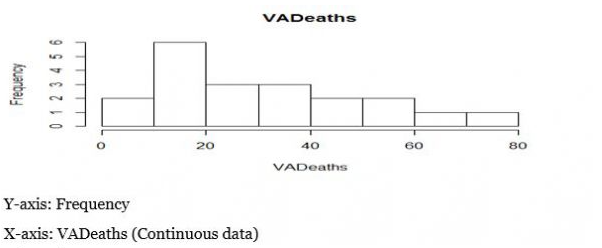Coloured Histogram:
>install.packages(“RcolorBrewer”)
>library(RcolorBrewer)
>help(brewer.pal) #Creates colored bins
>par(mfrow=c(3,3))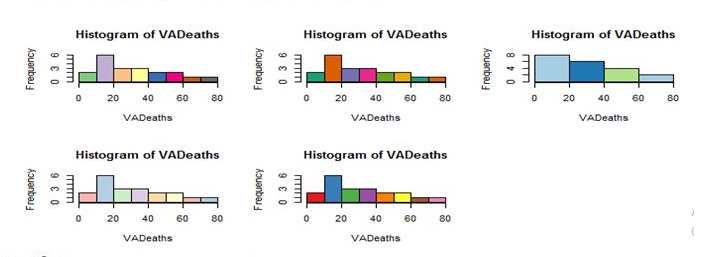Bar Plot:
Bar plot helps us in comparing cumulative totals across different variables.
Bar plot for single variable is a plot between magnitude of variables and its index
Bar Plot for Single Variable:
For Continuous data, bar plots simply show rectangular bars( length of each bar represents the magnitude of the variable).
>data(mtcars)
>barplot(mtcars&cyl,cor=brewer.pal(6,”set3”), xlab= “Index”,
ylab=”Cylinders, main=Cylinderdistribution)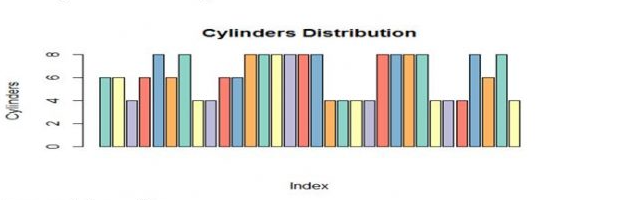For categorical type of data
>table(mtcars&cyl)
MTcars\$cyl
4    6    8
11    7    14

>barplot(table(mtcars\$cyl),col=brewer.pal(3,”Dark2”),
names.arg=c(“4”,”6”,”8”),xl ab=”cylinder type”, ylab=”count of cylinder”)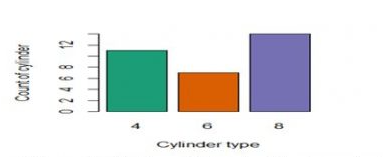2 different visualizations with bar plots( For Comparing the cumulative totals across different variables)

Stacked bar Plot
>barplot(table(cyl,gear),names.arg=c(“3”,”4”,”5”),xlab=”GearType”,
ylab=”Count of Cylinders”,
col=brewer.pal(3,”Dark2”);legend(“topright”,c(“4”,”6”,”8”),
fill=brewer.pal(3,”Dark2”),cex=1)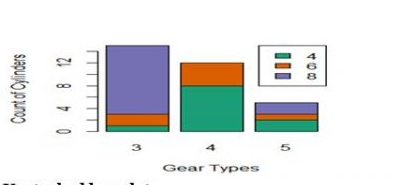Unstacked Barplot
>barplot(table(cyl,gear),names.arg=c(“3”,”4”,”5”),xlab=”GearType”,
ylab=”Count of Cylinders”,
col=brewer.pal(3,”Dark2”),beside=T);legend(“topright”,c(“4”,”6”,”8”),
fill=brewer.pal(3,”Dark2”),cex=1)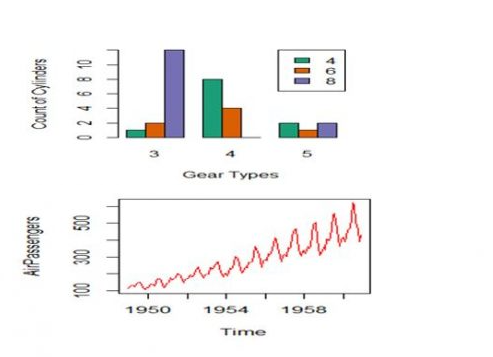Box Plot
Box plot is used for visualizing the spread of data and to detect outliers if any, it is also useful to know if data is skewed or not.
When a box plot is plotted, the below mentioned five statistical values are displayed
-Minimum
-1st quartile(median of 1st 50% of data)
-Median line
-3rd quartile(median of last 50% of data)
-Maximum
InterQuartile Range(IQR) is the spread of the data that is present inside the rectangular box of box plot

Box plot visualization:
>data()
>libraries(datasets)
>data(mtcars)
>data(Airpassengers)
>data(iris)
>par(mfrow=c(2,2)
>boxplot(mtcars\$mpg,col=”green”, ylab=”MPG”)
>boxplot(iris\$Sepal.Length ~ iris\$Species,col=brewer.pal(9,”Dark2”,
xlab=”Species”, ylab=”sepal length”)
>boxplot(mtcars\$mpg ~ mtcars\$gear,col=brewer.pal(4,”Accent”,xlab=”GearType")
>bloxplot(AirPassengers~cycle(Airpassengers),col=brewer.pal(12,”Dark2”),
xlab=”Month”, names=c( “Jan”, “feb”, “Mar, “April”, “Jun”, July, Aug”,
“Sep”, “Oct”, “Nov”, “Dec”)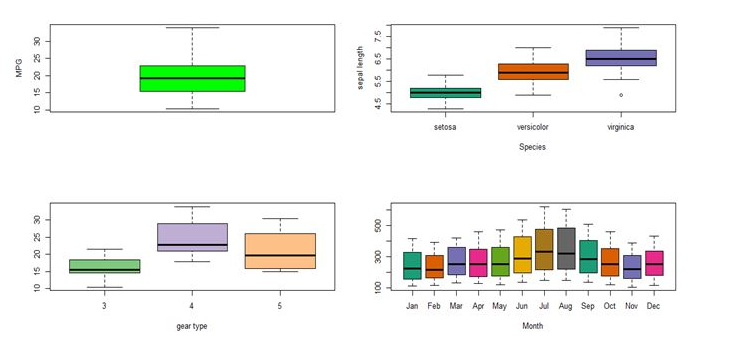Scatter Plot:
For Single Variable, it is a visualization, which is very simple and it talks about the spread of data
If the scatter plot is plotted between 2 variables then it shows the correlation between those 2 variables.
Scatter plot for a single variable
>plot(iris\$Sepal.Lenghth,xlab = “index”, ylab=”sepal length”, col=”red”,
type=”o”)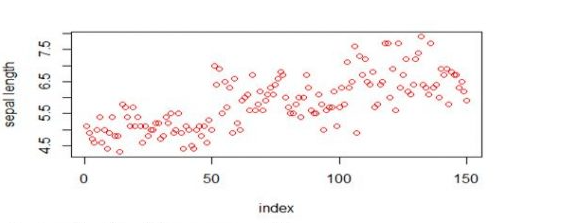Scatter plot for 2 variables
>plot(iris\$Sepal.Lenghth, iris\$Sepal.Width,xlab=”sepal length”,
ylab=”sepal Width”, col=”blue”, type=”p”)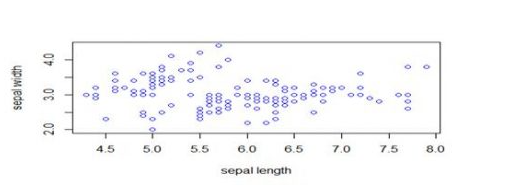Scatter plot matrix will help visualize the variables each other
>Plot(iris,col=brewer.par(10,”Dark2”))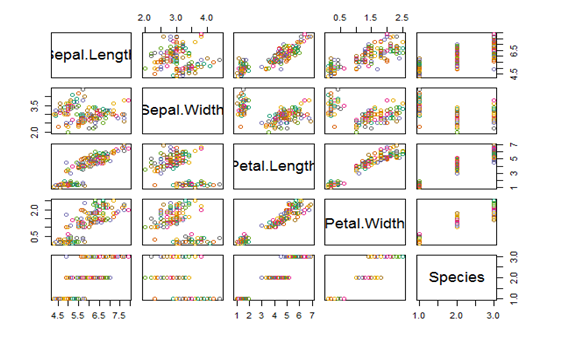Pie chart
>pie(table(iris\$Species),col=brewer.pal(3,”Dark2”))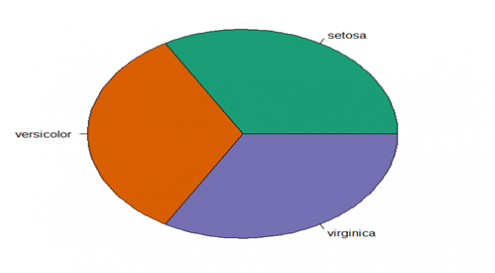3D pie chart
>install.packages(“plotrix”)
>library(plotrix)
>pie3D(table(iris\$Species),labels=c(“setosa”, “versicolor”,
virginica”),explode=0.2)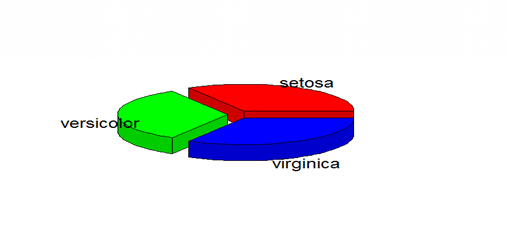Correlogram:
These are used to test the level of correlation among the variables present in the dataset.
The cells of the correlogram are shaded or colored to show the correlation value.
Darker the color, higher is the correlation between the variables.
>data(mtcars)
>install.packages(“corrgram”)
>library(corrgram)
#Normal Correlogram Plot
>corrgram(mtcars)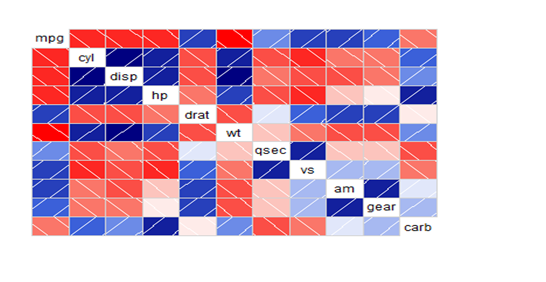#Correlogram with scatter plot between variables
>corrgram(mtcars,lower.panel=panel.cor,upper.panel=panel.pts)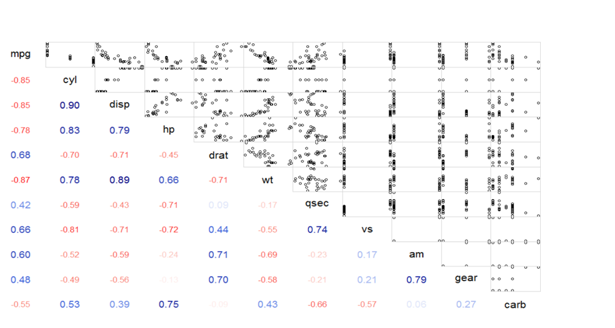#Correlogram with correlation values and pie plot
>corrgram(mtcars,lower.panel=panel.pie,upper.panel=panel.pts)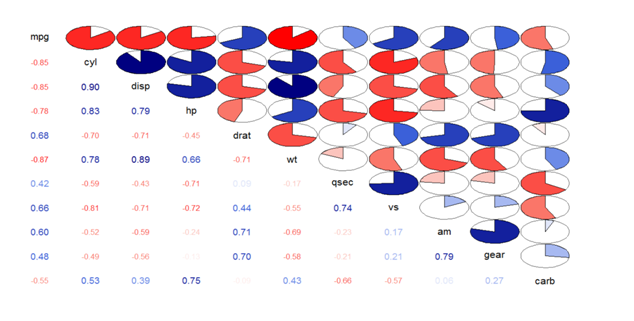#Correlation and correlogram plot
>install.packages(“corrplot”)
>library(corrplot)
Cor_matrix<-corr(mtcars)     #Correlation values between variables
>corrplot(cor_matrix)         #Normal Correlation correlogram plot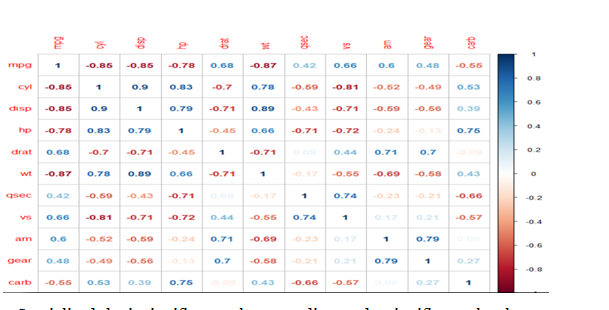#Specialized the insignificant values according to significance level
col=brewer.pal(5,”set2”))
#level=>level of administrative sub division (0 or 1 or 2)
>plot(c_ind_o,col=”lightgreen”, main=”INDIA”)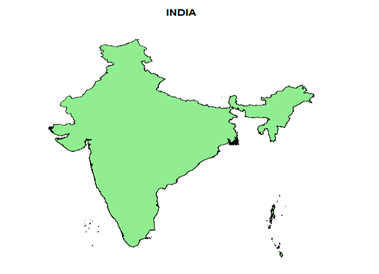#To Display States
>library(RcolorBrewer)
>plot(c_ind_1,col=brewer.pal(12,”Dark2”),main=”INDIA”)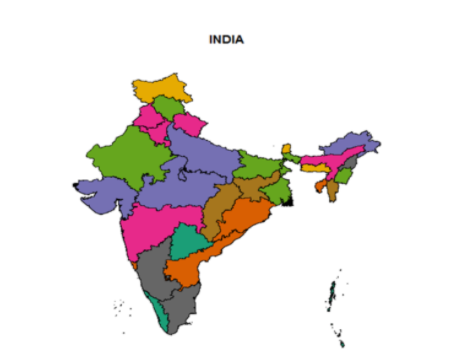>c_ind_2_maharasthra<- subset(c_ind_2,c_ind_2\$Name_1== “Maharashtra”)
>plot(c_ind_2_maharasthra,col=brewer.pal(12,”Dark2”))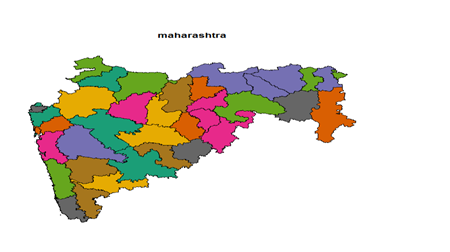>c_ind_1_maharasthra<- subset(c_ind_1,c_ind_1\$Name_1==”Maharashtra”)
>plot(c_ind_1_maharasthra,col=brewer.pal(12, “Dark2”))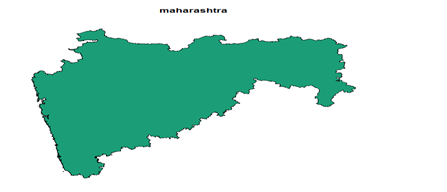#World Climate

#To get the unemployment rate of statesIndiana and Florida
Unemployment_Indiana_Florida<-
get_bls_county(statename=c(“Indiana”,”Florida))

>bls_map_county(map_data=df,fill_rate = “unemployed_rate”,labtitle = “unemployment Rate in Indiana”,highFill = “blue”,lowFill = “green”,stateName = c(“Indiana” , “Florida”))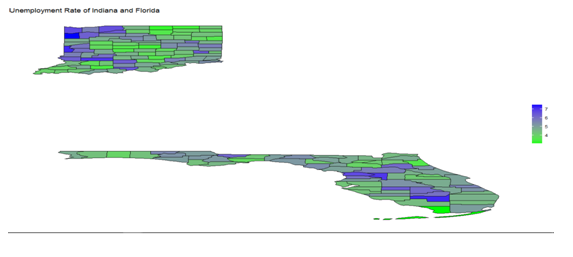# To display correlation coefficient between any 2 variables
>corrplot(cor_matrix,method= “number”)